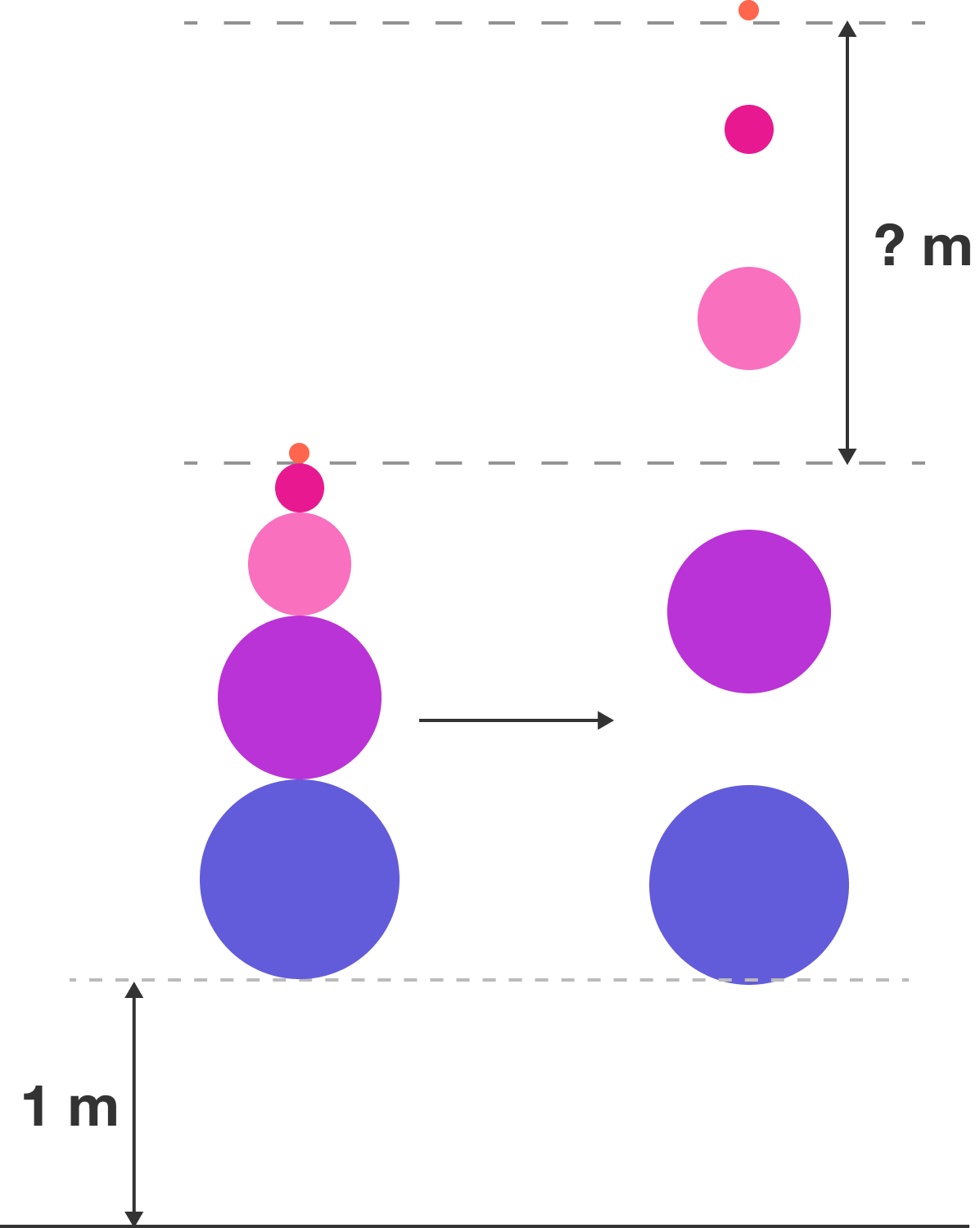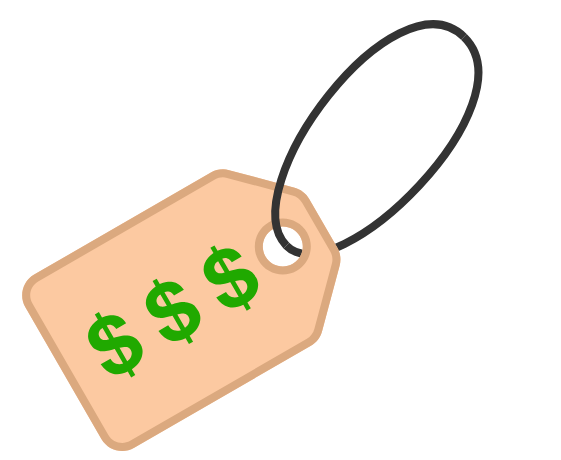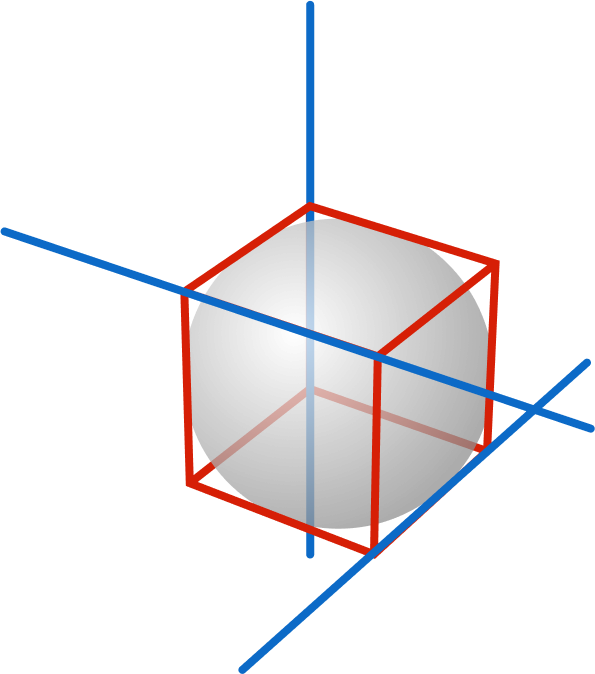# Problems of the Week

Contribute a problem

$d_n$ is the last nonzero digit of the decimal representation of $n!$.

Is $d_n$ eventually periodic?

Clarification: $d_n$ is called "eventually periodic" if there exist $k$ and $n_0$ such that $d_{n+k}=d_n$ for all $n\geq n_0.$

Harry stacks 5 balls one on top of another, raises them so that the bottom ball is $\SI{1}{\meter}$ off the ground, and drops them as shown in the diagram. He has the freedom to set the masses of the five balls to whatever values he pleases.What is the least upper bound of the height to which the top ball can rise above its starting position (in $\si{\meter}$)?

Assumptions:

• All collisions are perfectly elastic and there is no air resistance.
• You may want to try this related problem as a warmup.
• The figure is not drawn to scale.

Consider the binary look-and-say sequence: $1,{\color{#D61F06}1}1,{\color{#D61F06}10}1,{\color{#D61F06}1}1{\color{#D61F06}1}0{\color{#D61F06}1}1,{\color{#D61F06}11}1{\color{#D61F06}1}0{\color{#D61F06}10}1,$ and so on.

Let $f(n)$ denote the times the string "100" appears in the $n^\text{th}$ term. Then $f(8)=2,$ for example, because the $8^\text{th}$ term equals $111{\color{#D61F06}100}111010110{\color{#D61F06}100}110111011.$

What is $\displaystyle\lim_{n\to\infty}\frac{f(n)}{f(n-1)}?$While shopping, you find an item with a random price evenly distributed between $0 and$60. You buy as many of these items as possible with the $60 you brought. What is the expected amount of money you have spent (in dollars)? Note: You may assume that$60 is large compared to a penny so that the distribution of prices is essentially continuous.Given a unit cube, extend 3 of its edges into non-intersecting, non-parallel lines (in blue) in 3D space. Then five and only five spheres of the same radius are each tangent to all 3 of the lines.

What is the radius of each of these spheres?

The figure at right illustrates a sphere tangent to the 3 blue lines, but there can only be one such sphere with that radius. (Remember, we need five identical spheres satisfying the tangency condition!)

Suppose the radius of each of the five spheres can be expressed as $\frac{a}{b}\sqrt{c},$ where integers $a$ and $b$ are coprime and integer $c$ is square-free.

What is the product $a \times b \times c?$

Note: Spheres may intersect one another.

×